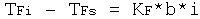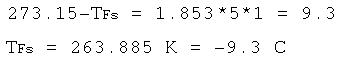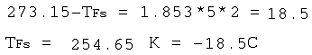Freezing Point Depression: Definition, Equation & Examples

Instructor: Laura Foist

Laura has a Masters of Science in Food Science and Human Nutrition and has taught college Science.

In this lesson, we will describe what it means to have a freezing point depression. We will explain how we determine the new freezing point and show examples of when this is used in the world.

Freezing Point Depression

On a hot summer day it is fun to enjoy a nice refreshing ice cream cone. Or you may prefer a simple glass of water with ice in it. Did you know that the temperature of the ice cream is colder than the temperature of the ice? Even though they are both frozen and both have 'ice' in the name, the temperatures are different.

The freezing point can vary based on what solutes are in a liquid; this is called freezing point depression. If water has salt in it, then the freezing point would be lower than just pure water. This has many practical uses such as why we salt the roads in winter and how we make ice cream.

Equation

In order to determine the new freezing point of a solution (Tfs) we first need to know some information. First we need to know the freezing point of the solvent (Tfi). So, for water, we know that the freezing point is 0 degrees Celsius (or 32 degrees Fahrenheit). We also need to know the molality (b). Molality is moles per kilogram, so it is the number of moles of solute (such as salt) per kilogram of solvent (such as water).

We also need to know the Van't Hoff factor (i). The Van't Hoff factor is determined based on how many ion particles are in the solution. Since some compounds will separate in solution it acts like 2 or more individual molecules. For example, salt (NaCl) will separate into sodium ions and chlorine ions, so the Van't Hoff factor for salt is 2. But a molecule such as sugar doesn't separate even in solution, so the Van't Hoff factor is 1.

The last thing we need to know is the cryoscopic constant (Kf). The cryoscopic constant is different for every solvent since it depends on the properties of the solvent. For example, the cryoscopic constant for water equals 1.853 K*kg/mol, and for acetic acid (vinegar) it is 3.90 K*kg/mol. The K is an abbreviation for Kelvin, which is a unit of measure for temperature.

The equation is:So, if you have 5 moles of sugar (which equals 1711.5 grams of sugar in 1 kg of water) dissolved in water, we can determine the new freezing point as long as we know the freezing point of pure water. In order to keep the same units we need to use it in Kelvin, which is 273.15 K.We end up with 263.885 K, which is equal to -9.3 degrees Celsius. So, the sugar decreases the freezing point of the water by nearly 10 degrees.

Now, let's see how salt changes the freezing point of water. Let's again use 5 moles of salt (which equals 292 grams of salt in 1 kg of water).We end up with 254.65 K, or -18.5 degrees Celsius. Since the salt separates into two separate units it decreases the freezing point by a lot more than the sugar does.

Examples

We use this concept of lower freezing points in a lot of real world examples. Two that you probably frequently see are in ice cream making and salting the roads in the winter.

To unlock this lesson you must be a Study.com Member.

Register to view this lesson

Are you a student or a teacher?